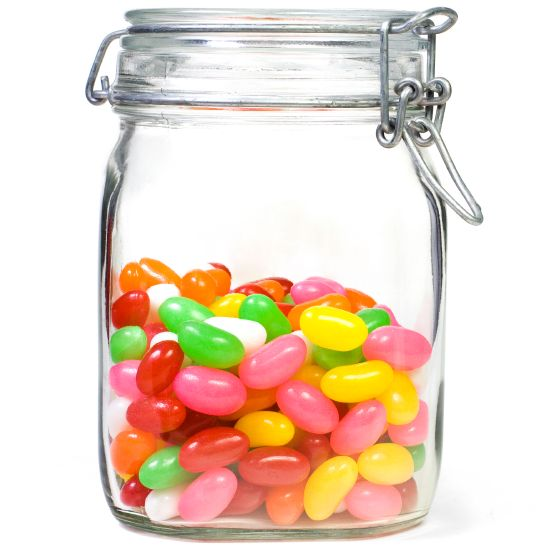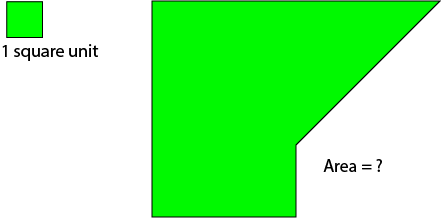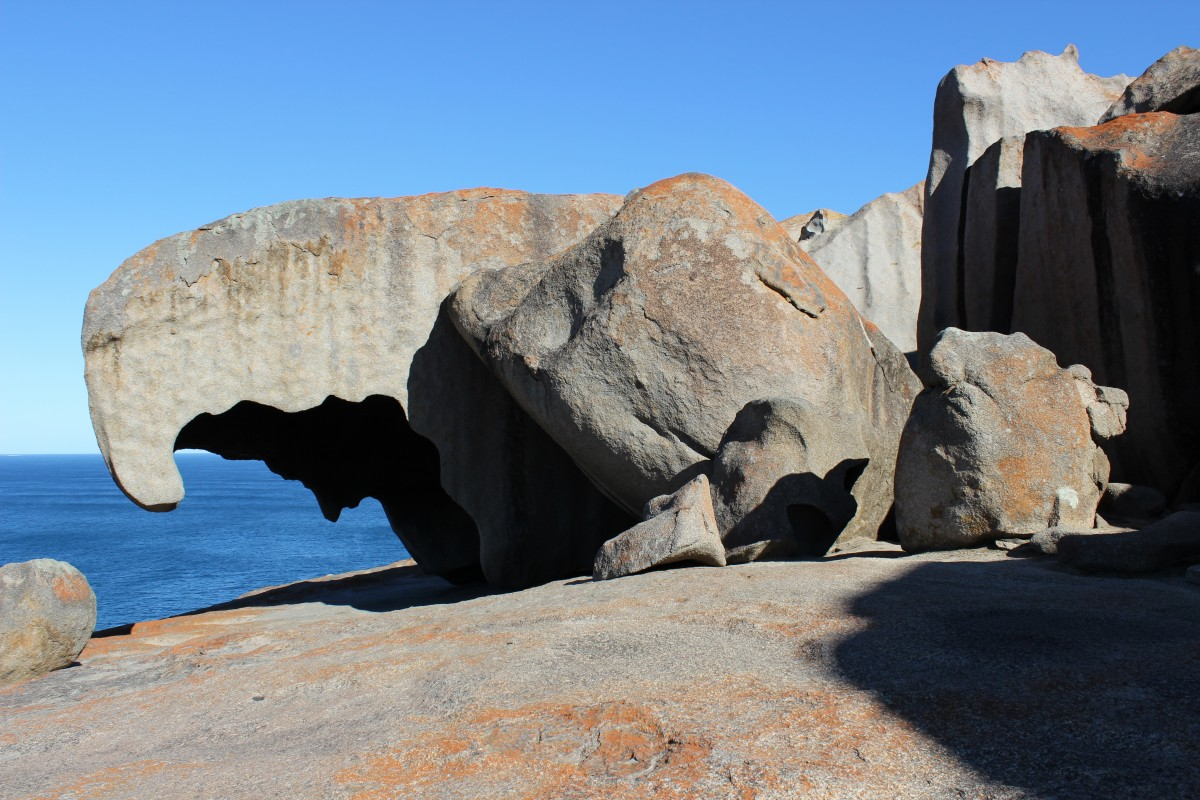# Visual Estimation

Estimation is a very important skill. When we estimate we are not looking for an exact answer. We're trying to get an answer that is close enough to the right answer so that it will give us a good idea of what the right answer should beHave you ever entered a jelly bean guessing competition? If you haven't, the idea is to guess the number of jelly beans in a jar. Each person writes down their guess, and the person with the guess that's closest to the number of jelly beans in the jar wins the jar of jelly beans.

Visual estimation can help you to guess the number of jelly beans in the jar, and give you a good chance of winning the competition. For example, you could estimate the number of jelly beans on one layer in the jar, then estimate the number of rows. Multiplying these two numbers together will give you a good estimate for the total number of jelly beans in the jar. Maybe you think there are $25$ jelly beans on each layer, and $7$ layers of jelly beans in the jar. Your visual estimate would give you a guess of $7 \times 25 = 175$ jelly beans in this jar. This might be good enough to win the competition.

Did you notice how we used our brain as well as our eyes to come up with that visual estimate? Visual estimation always involves thinking as well as looking.

## Estimating Things Based on Something You Know

Sometimes we can use something we know about to help us to come up with a good visual estimate for something else. For example, we know that the small square in the picture below has an area of $1$ square unit. We can use this information to estimate the area of the larger shape.Let's divide the larger shape up into a rectangle and a triangle (just extend that short vertical side up to the top of the shape). It looks like the small square will fit along the vertical edge of the rectangle six times, and across its width about 4 times. So, the area of the rectangle is about $6 \times 4 = 24$ square units. Now we need to estimate the area of the triangle. It looks as if it's half of a square. The small square would fit along the sides of this bigger square four times each, so the area of square would be $4 \times 4 = 16$ square units, and the area of the triangle would be about half of that, or $8$ square units. The total area of the larger shape would then be about $24 + 8 = 32$ square units.

If you know the usual size of things, it can help you to make estimates of distances, or the sizes of other things.

Estimation gives you a quick answer that is near enough to the correct answer. It does not give the exact answer that you can sometimes get by doing calculations.

## Estimating Things Other Than the Results of Calculations

We can use estimation for things other than the results of calculations. It's Christmas time now, so I've been wrapping lots of presents. It has been very handy to be able to estimate the amount of paper I need to wrap each parcel. If you're planning a party, it's really useful to be able to estimate the number of people that would fit in a room, or the size of slices you should cut from your birthday cake so that everyone gets a fair share. You might need to estimate the amount of material you need to make a costume, or the number of litres of paint that are needed to paint your house.

When you're out bush walking, it's really handy to be able to make visual estimates of distances.You can use your thumb to estimate how far away something is, provided you know how big that thing is. Suppose you are out walking and you spy the rocks in the picture. The rock that looks like a bird's beak is about $12$ metres wide. You can use your thumb to work out how far away it is:

• Close one eye and hold your arm out in line with the rock with your thumb sticking straight upwards
• Open that eye and close the other one, while still keeping your thumb in the same position.
• Estimate how far (on the rock) your thumb seemed to move sideways when you changed eyes.
• Multiply the changed distance by $10$
So, if your thumb seemed to move about $\dfrac{1}{4}$ of the width of the rock (3 metres), then the rock must be about $10 \times 3 = 30$ metres away from you.## How do I get good at estimating things?

I'm glad you asked! Like any skill, the only way to get good at estimation is to do lots of practice:

• Try and estimate distances using your thumb when you're out walking. Check your estimates using paces.
• When you have to calculate areas and perimeters at school, try to estimate them visually at first. Use a square of a known area or a line of a known length to base your estimates on.
• Practise for that jelly bean guessing competition. Try to visually estimate the numbers of objects in groups whenever you see a lot of objects together.

### Description

This mini book covers the core of Math for Foundation, Grade 1 and Grade 2 mathematics including

1. Numbers
3. Subtraction
4. Division
5. Algebra
6. Geometry
7. Data
8. Estimation
9. Probability/Chance
10. Measurement
11. Time
12. Money
13. and much more

This material is provided free of cost for Parent looking for some tricks for their Prekinder, Kinder, Prep, Year 1 and Year 2 children

### Learning Objectives

These lessons are for kids aged 4-8 with the core objective to expose their brains to concepts of addition, subtraction, division, algebra and much more.

Author: Subject Coach
You must be logged in as Student to ask a Question.